# Printable Hundred SquareUpdated on May 27, 2021By Printablee Team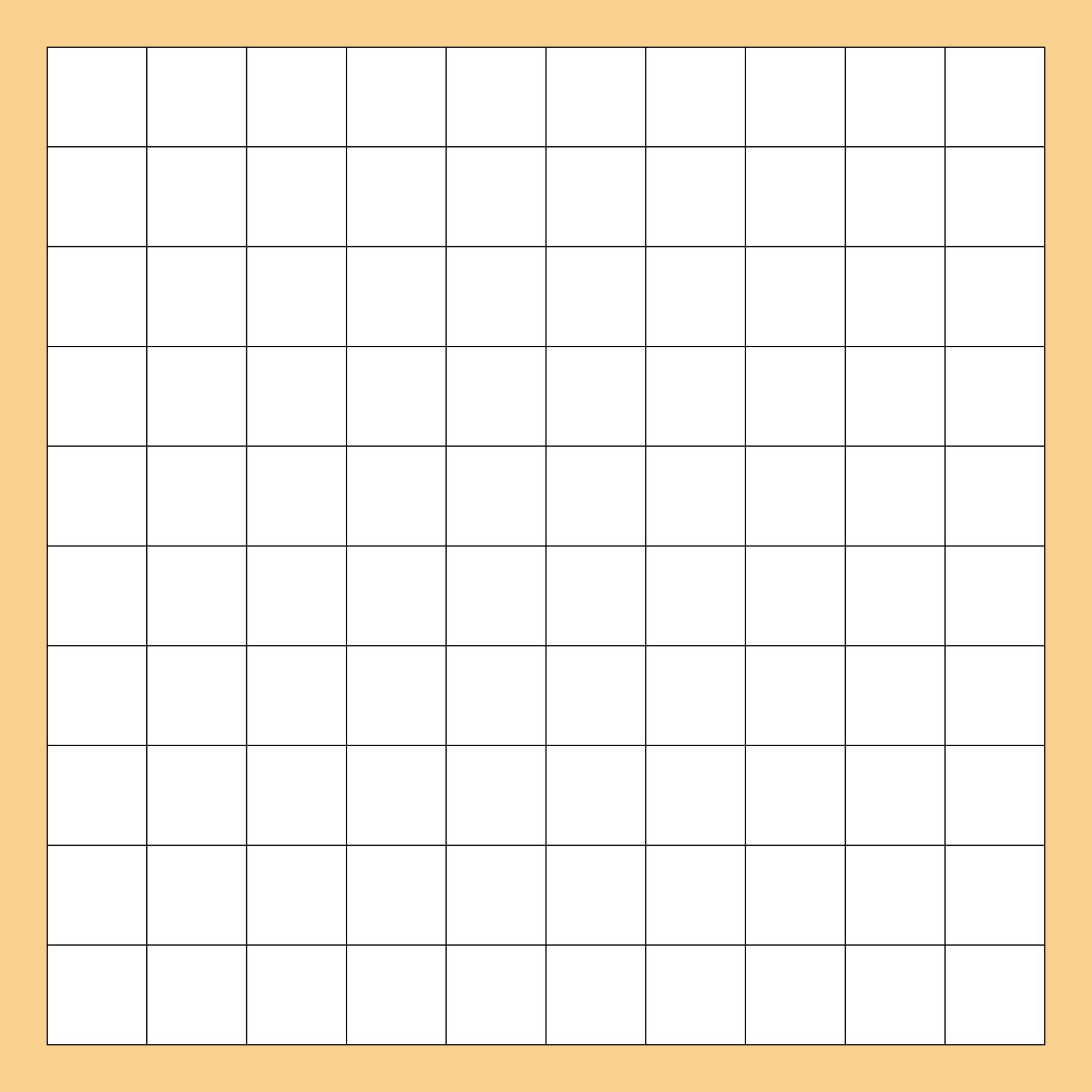### How to memorize multiplication with a hundred square?

Multiplication can be tricky and difficult for some children. Not all children are able to solve or understand Math easily. Therefore we need a tool that can help him. With a hundred square which has a function to be filled by a row of numbers, you can use it to help children remember multiplications. However, before using the hundred square, children must be given a basic understanding of multiplication. After that, it can be continued with various tools that can help children remember, for example, using a hundred square. This tool can stimulate children's memory with a visual hundred square.

### Can I make a multiplication chart with hundred square?

The use of the hundred square as a useful tool in Math is not something new. One of the basic operations in Math that can use a hundred squares is multiplication. Usually, multiplication charts come in many forms but when it comes to a hundred squares, of course, you can use them as multiplication charts. The hundred square only needs to be filled in with the compiled numbers according to the type of multiplication that you want to make into the chart. Usually, at a hundred square you can have 10 types of multiplication.

### Can I do colouring in a hundred square?

Using a touch of colour on a hundred square can help maximize its use. With the many colours in a hundred square, you can see a pattern forming in each square. However, giving colour can not be done carelessly because it can leave a random impression and does not provide a pattern that matches the placement of the numbers. For example, if you want to create multiplication charts on a hundred square, the colour selection can be adjusted according to each type of multiplication that is in it.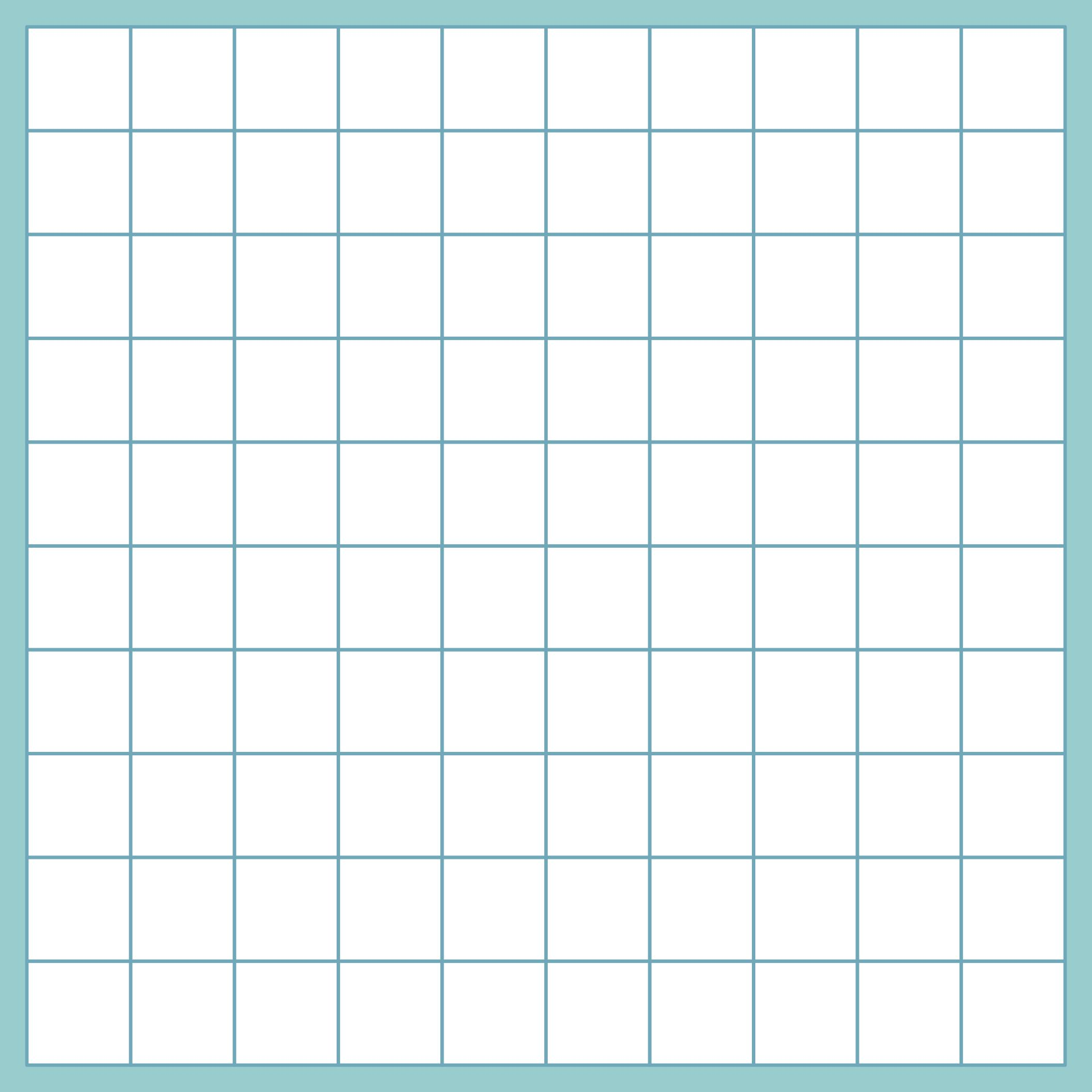We also have more printable other you may like:
Printable 100 Square Grid
Super Bowl Football Squares Printable
Printable Bingo Dauber Dot Activities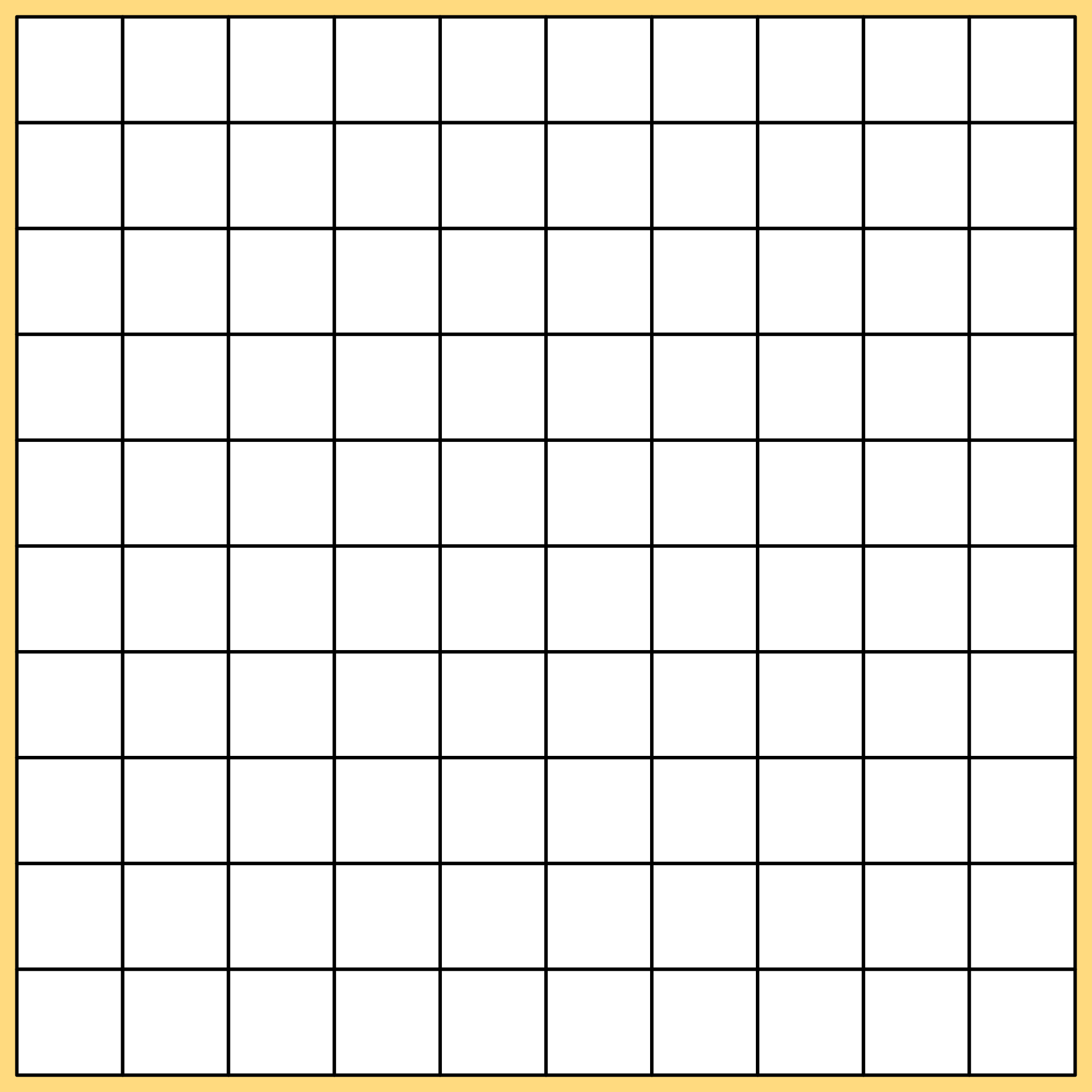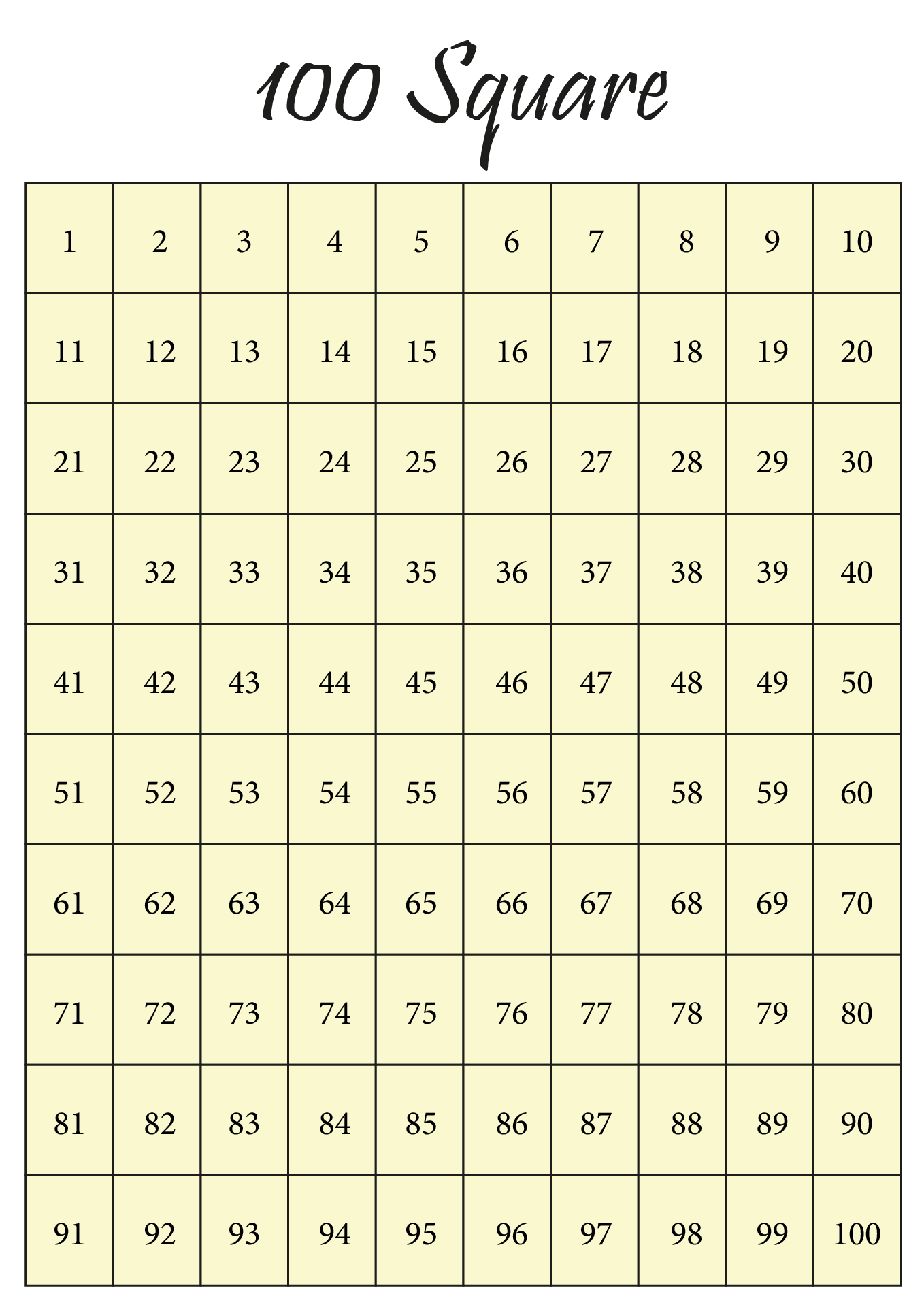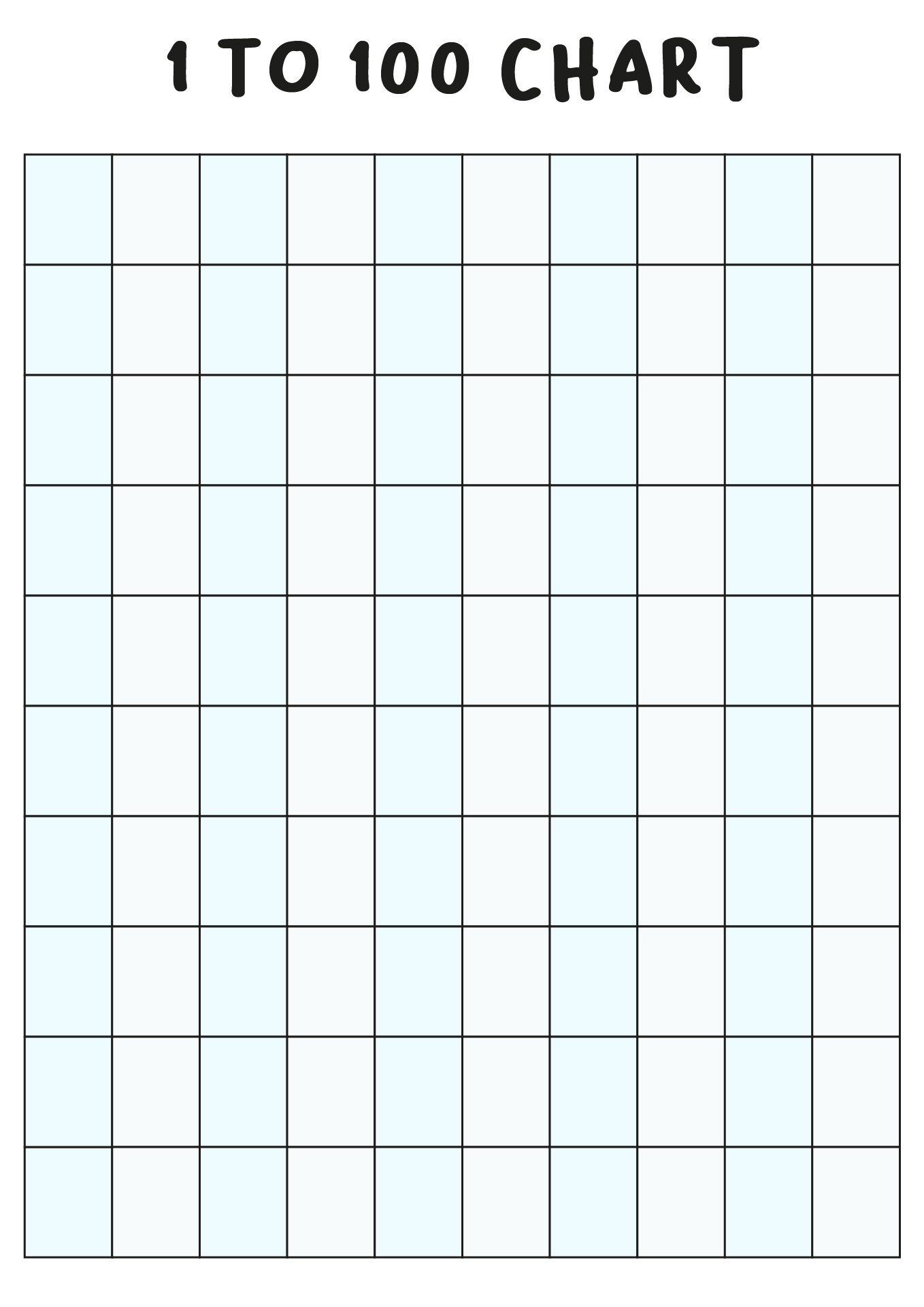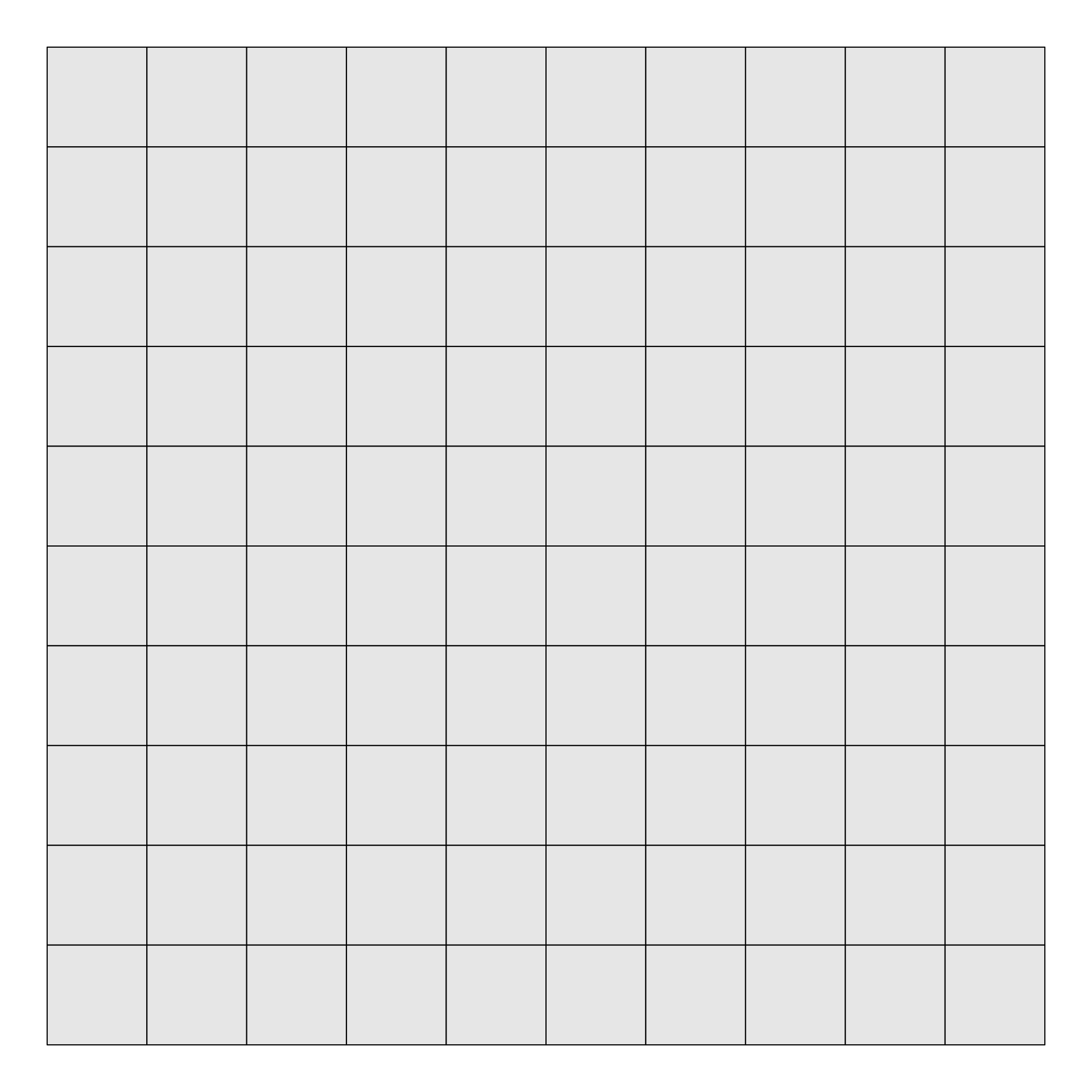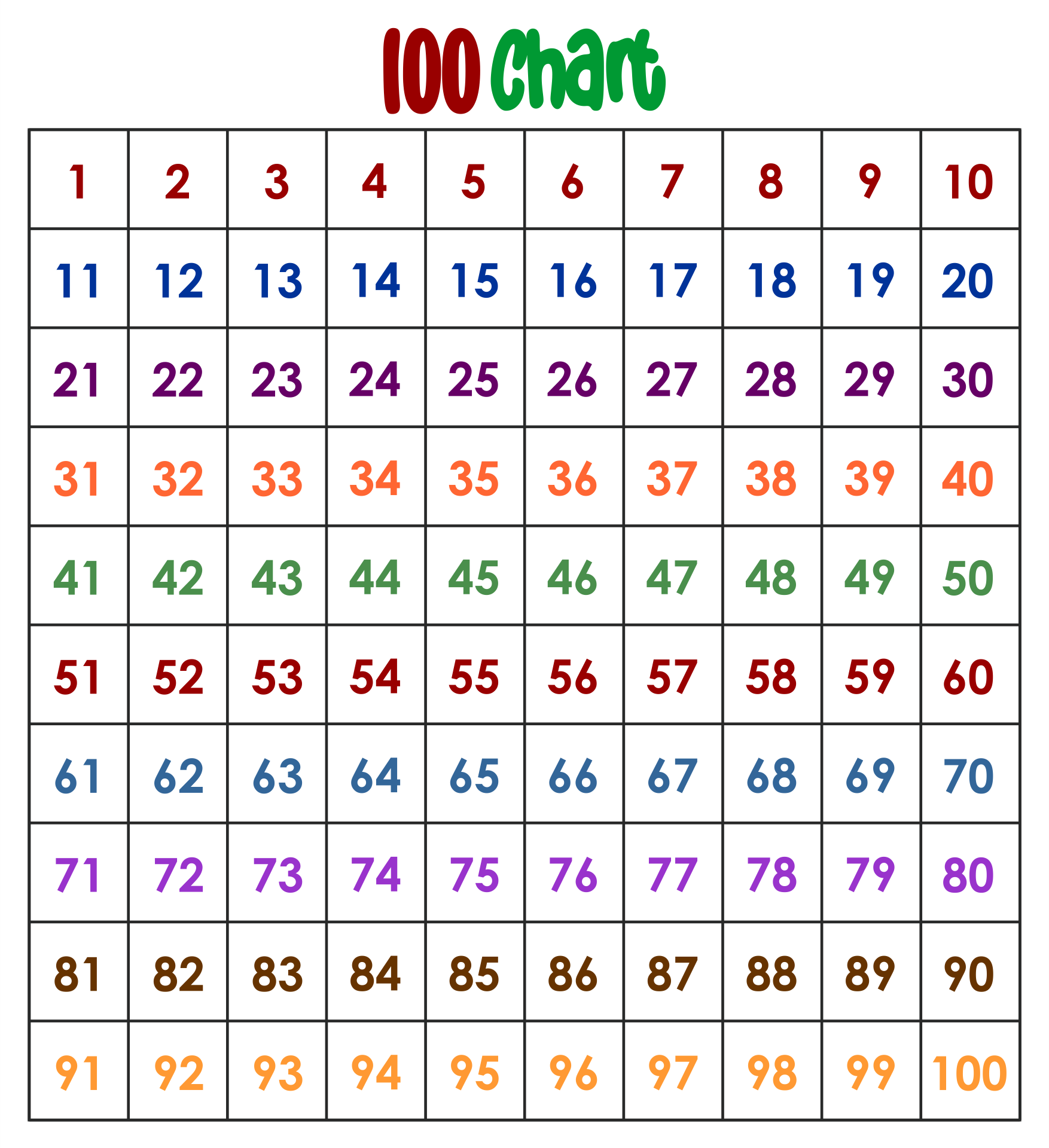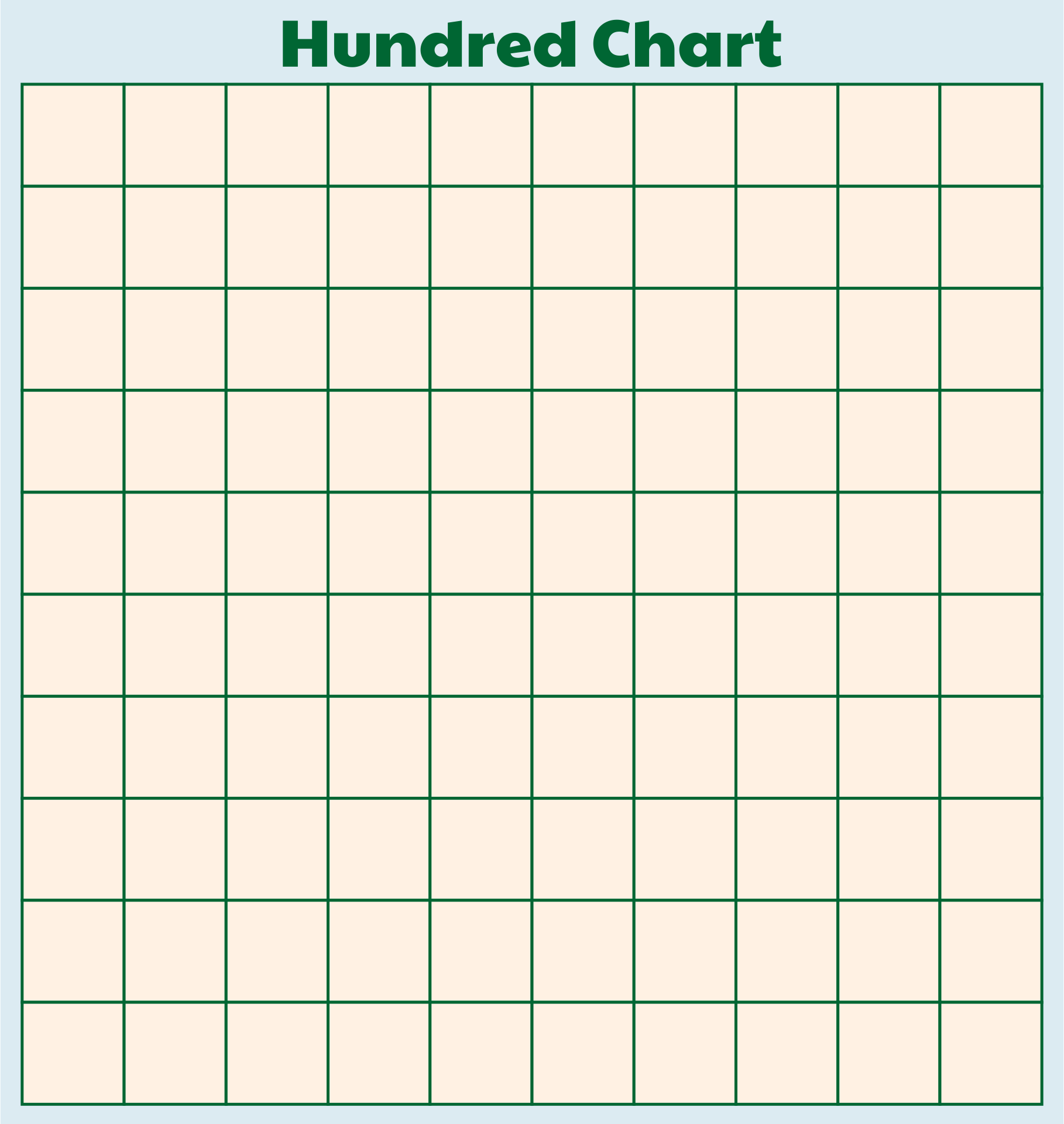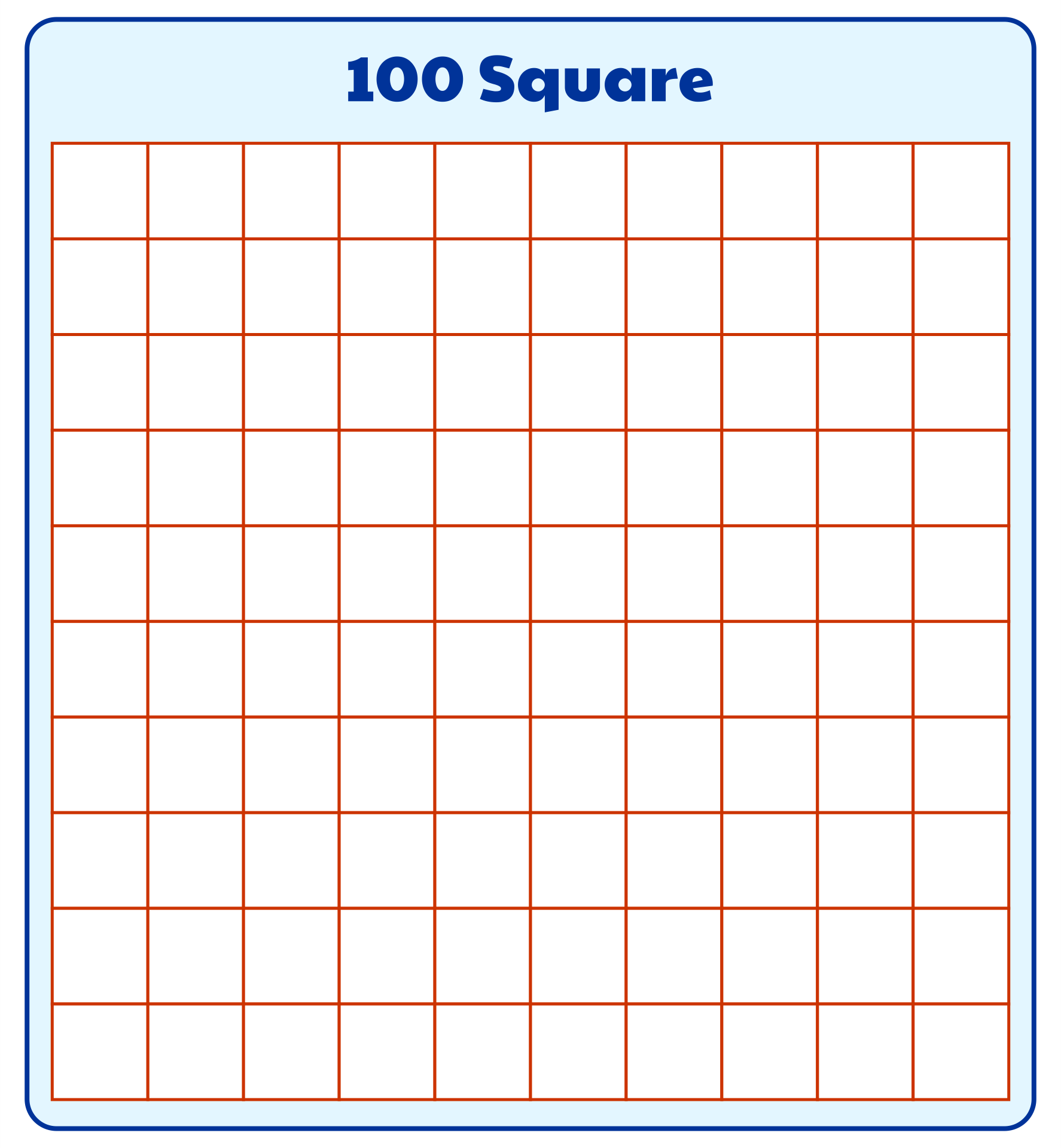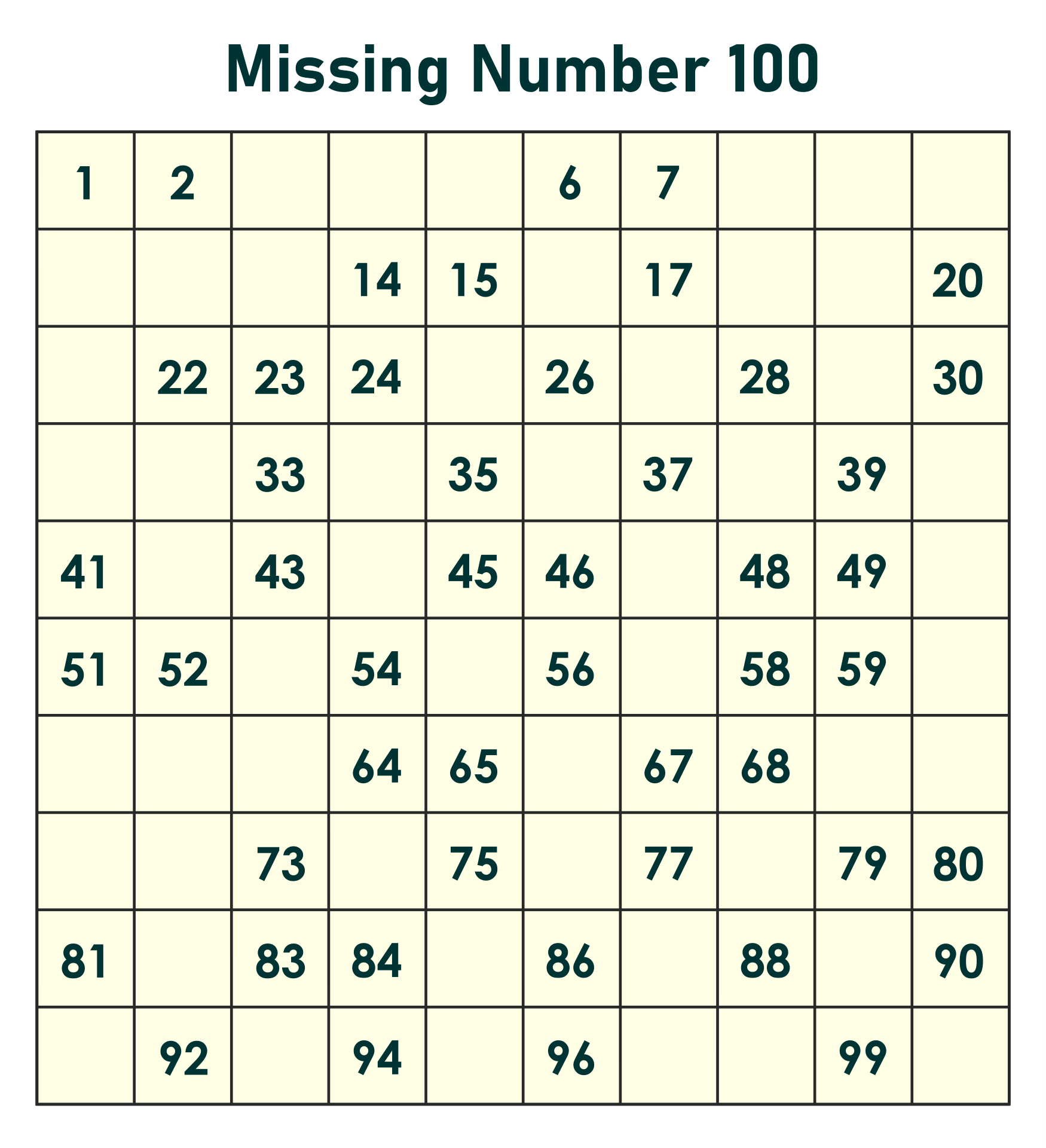### How to make a hundred square more interesting?

Hundreds of squares can be really interesting if you know to put the fun in it. Not only on the basic calculation of Math or something serious but it can also be used for other fun activities, one of which is games. There are lots of games that require grids, squares and something like a hundred square. The colouring activity as mentioned earlier also becomes something fun to make hundreds square more interesting. You can also add decorations or choose a container other than paper to make a hundred square. Doing DIY exploration with a hundred squares can be an option to do.